# Algebra Terms Flashcards

Algebra Terms Flashcards
1/25 (missed) 0 0
Create Your Account To Continue Studying

As a member, you'll also get unlimited access to over 79,000 lessons in math, English, science, history, and more. Plus, get practice tests, quizzes, and personalized coaching to help you succeed.

Try it risk-free for 30 days. Cancel anytime
What is the constant in this equation: 6 - 2x?
The constant is 6.
Got it
What is the constant in the equation 2x + 5?
The constant is 5.
Got it
What are the terms in this equation: 2x + 6 = 18?
The terms are 2x, 6, and 18
Got it
What is the coefficient in this equation 3x + 4 = 16?

The number '3' is the coefficient.

Got it
In the equation, 4 - y = 8, what is the variable?
y is the variable.
Got it
In the equation, x + 3 = 7, what is the variable?
x is the variable.
Got it
Define the following term: Constants

Terms that are just numbers.

Got it
Define the following: Terms

Groups of coefficients and variables.

Got it
Define the following term: Coefficients

The numbers in front of, or multiplying, the variables.

Got it
What is the most common letter used in algebra?
x
Got it
Define the following term: Variables

The letters in an equation.

Got it
Define the following term: Equation

Something equals something; look for the equal (=) sign.

Got it

or choose a specific lesson: See all lessons in this chapter
25 cards in set

## Flashcard Content Overview

This flashcard set covers some basic vocabulary terms in algebra, including variable, coefficient, term and constant. It will also help you visualize what these words mean in relation to the different parts of an algebraic equation. As you'll see these vocabulary terms many times during your algebra studies, it's important to know what they mean when approaching a set of directions or new material.

Algebra involves more than just numbers. It also includes working with letters and symbols. But what do those letters in an algebraic equation mean? Is the letter always the same? How do you distinguish between numbers that stand alone and numbers that stand next to letters in an equation? Most importantly, do you know what mathematical symbol transforms a group of numbers into an equation?

If some of these questions have left you scratching your head, don't worry; after studying this flashcard set, you will have a clear understanding of some basic algebra vocabulary terms.

## Studying With Flashcards

Problems and directions in algebra can be confusing since they have letters, symbols and vocabulary terms that may not be known. Study and quiz yourself on these terms until you understand their meaning and know how to apply them to problems or directions in your algebra studies. You can refer back to these flashcards on your mobile device, tablet or computer, which can make it easy to find a vocabulary term as soon as you need it.

Front
Back
Define the following term: Equation

Something equals something; look for the equal (=) sign.

Define the following term: Variables

The letters in an equation.

What is the most common letter used in algebra?
x
Define the following term: Coefficients

The numbers in front of, or multiplying, the variables.

Define the following: Terms

Groups of coefficients and variables.

Define the following term: Constants

Terms that are just numbers.

In the equation, x + 3 = 7, what is the variable?
x is the variable.
In the equation, 4 - y = 8, what is the variable?
y is the variable.
What is the coefficient in this equation 3x + 4 = 16?

The number '3' is the coefficient.

What are the terms in this equation: 2x + 6 = 18?
The terms are 2x, 6, and 18
What is the constant in the equation 2x + 5?
The constant is 5.
What is the constant in this equation: 6 - 2x?
The constant is 6.
Is this an equation?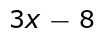No, because it does not have an equal (=) sign.
Is this an equation?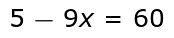Yes, because it has an equal (=) sign.
What are the constants in this equation?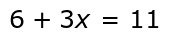6 and 11
What are the variables in this equation?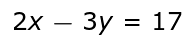x and y
What is the coefficient in the equation?2 and 3
What is the coefficient in the equation?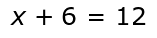1
What is the constant?-8
List the terms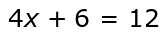4x, 6 and 12
is this an equation?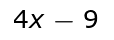No. There is no equal (=) sign.
List the terms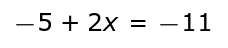-5, 2x and -11
Which letters can variables represent?
Variables can be any letter in the alphabet
Can variables have exponents such as this?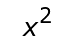Yes
Name the coefficient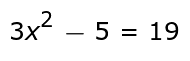3

To unlock this flashcard set you must be a Study.com Member.